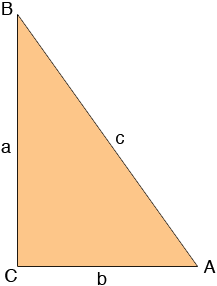SEARCH HOMEMath Central Quandaries & QueriesQuestion from jocelyn, a student: I wasn't able to solve this equation: only find the function using Pythagorean theorem Please help me.... sinA = 3/4 find secB tanA = 3/4 find cosA sinB = 4/5 find tan A cosA = 5 find csc A b =5; a= 12 find sin A c =25; a = 24 find cot A a = 6; c =10 find b? Find B when c = 25; a = 24 Find A when a = 5 and b = 12 csc = 1/2 find cosHi Jocelyn,

By your notation and the fact that you are to use the Pythagorean Theorem only I think you have a right triangle with angles A, B and C where C is the right angle and sides of length a, b and c as in the diagram below.If this is correct the the Pythagorean Theorem tell us that

a2 + b2 = c2

and hence if you know two of a, b and c you can find the third. For example if a = 9 and c = 15 then

92 + b2 = 152 or 81 + b2 = 225
Hence b2 = 225 - 81 = 144 and thus b = 12.

Similarly if you know the definitions of the trig functions you can solve for the trig functions in your examples. For example if sinA = 12/15 then a = 12 and c = 15. From the calculation above using Pythagoras Theorem you know that b = 12 and thus

secB = c/a = 15/9 = 5/3.

I hope this helps,
PennyMath Central is supported by the University of Regina and The Pacific Institute for the Mathematical Sciences.